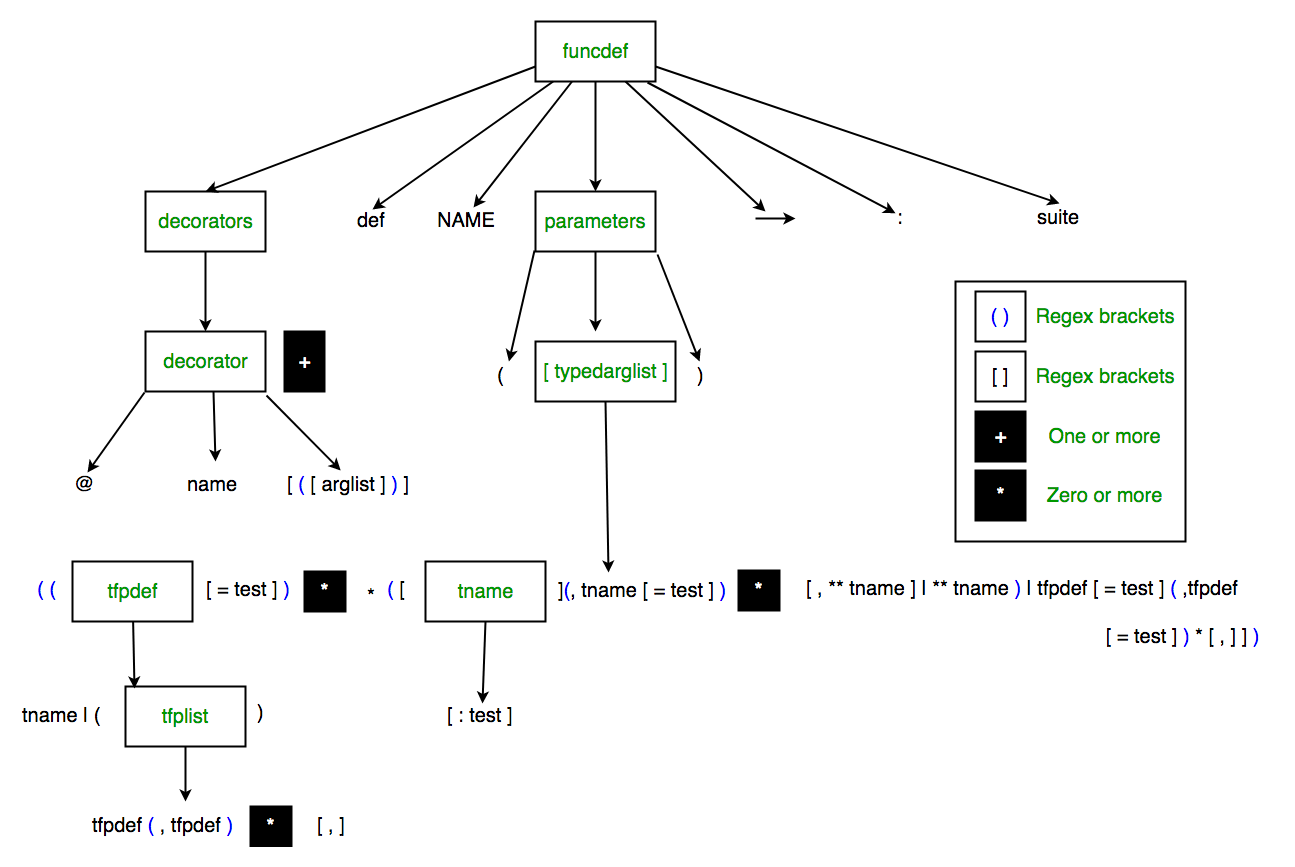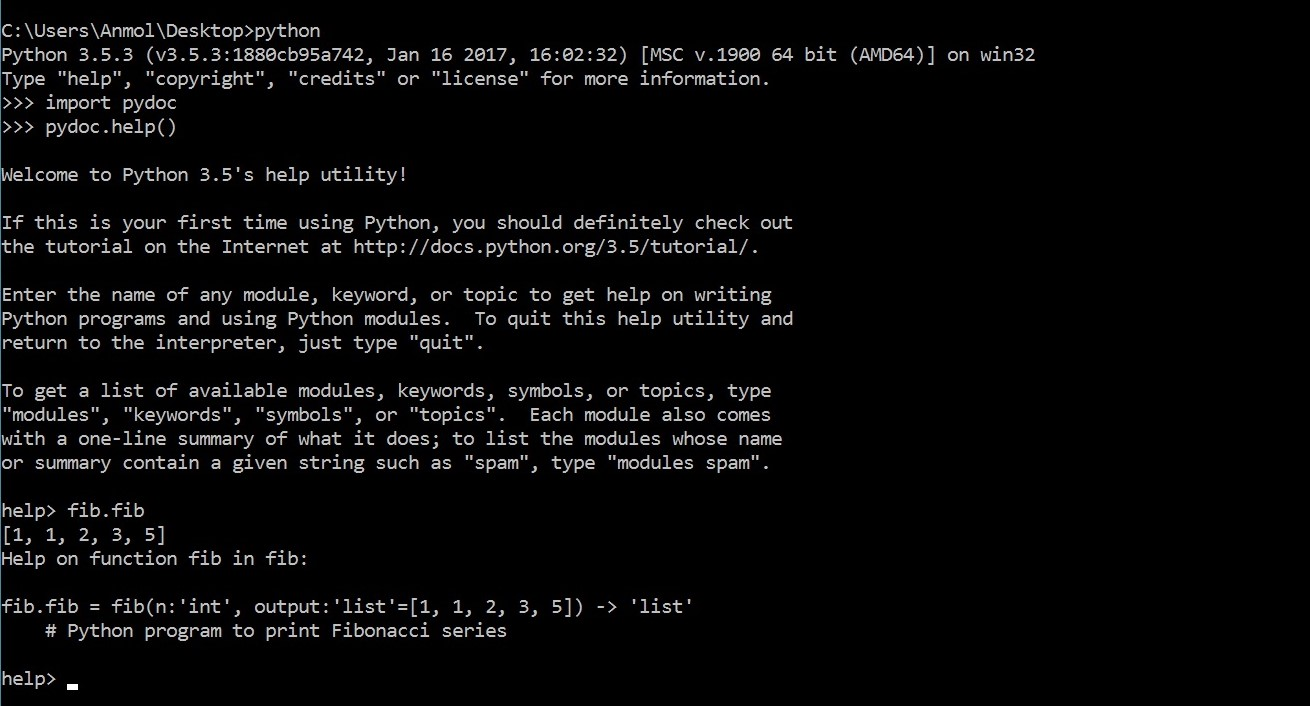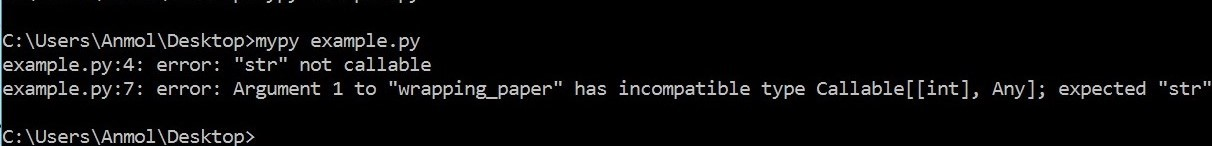Open in App
Not now

# Function Annotations in Python

• Difficulty Level : Hard
• Last Updated : 10 May, 2019

Basic Terminology

PEP: PEP stands for Python Enhancement Proposal. It is a design document that describes new features for Python or its processes or environment. It also provides information to the python community.
PEP is a primary mechanism for proposing major new features, for example – Python Web Server Gateway Interface, collecting the inputs of the community on the issues and documenting design decisions that have been implemented in Python.

Function Annotations – PEP 3107 : PEP-3107 introduced the concept and syntax for adding arbitrary metadata annotations to Python. It was introduced in Python3 which was previously done using external libraries in python 2.x

What are Function annotations?

Function annotations are arbitrary python expressions that are associated with various part of functions. These expressions are evaluated at compile time and have no life in python’s runtime environment. Python does not attach any meaning to these annotations. They take life when interpreted by third party libraries, for example, mypy.

Purpose of function annotations:
The benefits from function annotations can only be reaped via third party libraries. The type of benefits depends upon the type of the library, for example

1. Python supports dynamic typing and hence no module is provided for type checking. Annotations like
`[def foo(a:”int”, b:”float”=5.0)  -> ”int”]`

(syntax described in detail in the next section) can be used to collect information about the type of the parameters and the return type of the function to keep track of the type change occurring in the function. ‘mypy’ is one such library.

2. String based annotations can be used by the libraries to provide better help messages at compile time regarding the functionalities of various methods, classes and modules.

Syntax of function annotations

They are like the optional parameters that follow the parameter name.

Note: The word ‘expression’ mentioned below can be the type of the parameters that should be passed or comment or any arbitrary string that can be made use by external libraries in a meaningful way.

1. Annotations for simple parameters : The argument name is followed by ‘:’ which is then followed by the expression. Annotation syntax is shown below.
```def foobar(a: expression, b: expression = 5):
```
2. Annotations for excess parameters : Excess parameters for e.g. *args and **kwargs, allow arbitrary number of arguments to be passed in a function call. Annotation syntax of such parameters is shown below.
```def foobar(*args: expression, *kwargs: expression):
```
3. Annotations for nested parameters : Nested parameters are useful feature of python 2x where a tuple is passed in a function call and automatic unpacking takes place. This feature is removed in python 3x and manual unpacking should be done. Annotation is done after the variable and not after the tuple as shown below.
```def foobar((a: expression, b: expression), (c: expression, d: expression)):
```
4. Annotations for return type : Annotating return type is slightly different from annotating function arguments. The ‘->’ is followed by expression which is further followed by ‘:’. Annotation syntax of return type is shown below.
`def foobar(a: expression)->expression:`

Grammar

```decorator    :  ‘@’ name_  [‘(’ [arglist] ‘)’] NEWLINE
decorators   :  decorator+
funcdef      :  [decorators] ‘def’ NAME parameters [‘->’] ‘:’ suite
parameters   :  ‘(’ [typedarglist] ‘)’
typedarglist :  (( tfpdef [‘=’ test] ‘, ’)* (‘*’ [tname]
(‘, ’ tname [‘=’ test])* [‘, ’ ‘ **’ tname] | ‘**’ tname)
| tfpdef [‘=’ test (‘, ’ tfpdef [‘=’ test])* [‘, ’]])
tname        :  NAME [‘:’ test]
tfpdef       :  tname | ‘(’ tfplist ‘)’
tfplist      :  tfpdef (‘, ’ tfpdef)* [‘, ’]
```

Visualizing Grammar : The parse tree is formed from the above grammar to give better visualization of the syntax of the python’s function and function annotations.Sample Code

The code below will clear the fact that the function annotations are not evaluated at run time. The code prints fibonacci series upto the ‘n’ positions.

 `# Python program to print Fibonacci series``def` `fib(n:``'int'``, output:``'list'``=``[])``-``> ``'list'``:``    ``if` `n ``=``=` `0``:``        ``return` `output``    ``else``:``        ``if` `len``(output)< ``2``:``            ``output.append(``1``)``            ``fib(n``-``1``, output)``        ``else``:``            ``last ``=` `output[``-``1``]``            ``second_last ``=` `output[``-``2``]``            ``output.append(last ``+` `second_last)``            ``fib(n``-``1``, output)``        ``return` `output``print``(fib(``5``))`

`Output: [1, 1, 2, 3, 5]`

Note: Function annotations are only supported in python 3x.

Accessing Function Annotations

1. Using ‘__annotations__’ : The function annotations in the above code can be accessed by a special attribute ‘__annotations__’. It outputs the dictionary having a special key ‘return’ and other keys having name of the annotated arguments. The following code will print the annotations.

 `# Python program to illustrate Function Annotations``def` `fib(n:``'int'``, output:``'list'``=``[])``-``> ``'list'``:``    ``if` `n ``=``=` `0``:``        ``return` `output``    ``else``:``        ``if` `len``(output)< ``2``:``            ``output.append(``1``)``            ``fib(n``-``1``, output)``        ``else``:``            ``last ``=` `output[``-``1``]``            ``second_last ``=` `output[``-``2``]``            ``output.append(last ``+` `second_last)``            ``fib(n``-``1``, output)``        ``return` `output``print``(fib.__annotations__)`

```Output: {'return': 'list', 'n': 'int', 'output': 'list'}
```

2. Using standard module ‘pydoc’ : The ‘pydoc’ is a standard python module that returns the documentation inside a python module(if any). It has a special ‘help()’ method that provides an interactive shell to get help on any keyword, method, class or module. ‘help()’ can be used to access the function annotations. The image below shows the function annotations in the above Fibonacci series code. The module name is ‘fib.py’.3. Using standard module ‘inspect’: The ‘inspect’ module provides several useful functions to help get information about live objects such as modules, classes, methods, functions, tracebacks, frame objects, and code objects. We can use ‘getfullargspec’ method of the module to get complete information about the function which will contain the annotations.

 `# Python program to illustrate Function Annotations``import` `inspect``def` `fib(n:``'int'``, output:``'list'``=``[])``-``> ``'list'``:``    ``if` `n ``=``=` `0``:``        ``return` `output``    ``else``:``        ``if` `len``(output)< ``2``:``            ``output.append(``1``)``            ``fib(n``-``1``, output)``        ``else``:``            ``last ``=` `output[``-``1``]``            ``second_last ``=` `output[``-``2``]``            ``output.append(last ``+` `second_last)``            ``fib(n``-``1``, output)``        ``return` `output``print``(inspect.getfullargspec(fib))`

```Output: FullArgSpec(args=['n', 'output'], varargs=None,
varkw=None, defaults=([], ), kwonlyargs=[],
kwonlydefaults=None, annotations=
{'output': 'list', 'return': 'list', 'n': 'int'})```

Application of Function Annotations

• Use of ‘mypy’ : ‘mypy’ is an external library that provides static type checking with the help of function annotations.

`pip install mypy`

python 3x

`pip install git+git://github.com/JukkaL/mypy.git`

Example 1:

 `# String slicing function that returns a string from start index to end index.``def` `slice``(string:``str``, start: ``int``, end: ``int``) ``-``> ``str``:``    ``return` `string[start:end]`` ` `slice``([``1``, ``2``, ``3``, ``4``, ``5``], ``2``, ``4``)`

Save the above code as example.py and run the following command after installation of mypy. Make sure you are in the directory where you have saved the file.

`mypy example.py`

You will get the following result.• Things are little different when the decorators are involved.
Example 2(part a): Type checking of the parameters of the wrapped up function ‘gift_func’ and ‘wrapped’

 `def` `wrapping_paper(func):``    ``def` `wrapped(gift:``int``):``        ``return` `'I got a wrapped up {} for you'``.``format``(``str``(func(gift)))``    ``return` `wrapped`` ` `@wrapping_paper``def` `gift_func(giftname:``int``):``    ``return` `giftname``     ` `print``(gift_func(``'gtx 5000'``))`

At first, it may seem that passing string as an argument will return an error as the required datatype is an ‘int’ as annotated in ‘gift_func’ and ‘wrapped’. mypy does not establish typechecking in the wrapped up function parameters however typechecking of the decorator and the return type of the wrapped up functions can be checked. Therefore the following result can be expected from the above code.• Example 2(part b): Typechecking of the parameters of the decorator ‘wrapping_paper’.

 `def` `wrapping_paper(func:``str``):    ``    ``def` `wrapped(gift:``int``):``        ``return` `'I got a wrapped up {} for you'``.``format``(``str``(func(gift)))``    ``return` `wrapped`` ` `@wrapping_paper``def` `gift_func(giftname:``int``):``    ``return` `giftname``     ` `print``(gift_func(``'gtx 5000'``))`

You will now get the following result.• Example 2(part c): Typechecking of the return type of ‘gift_func’ and ‘wrapped’

 `# Suppose we want the return type to be int``from` `typing ``import` `Callable``def` `wrapping_paper(func):``    ``def` `wrapped(gift) ``-``> ``int``:``        ``return` `'I got a wrapped up {} for you'``.``format``(``str``(func(gift)))``    ``return` `wrapped`` ` `@wrapping_paper``def` `gift_func(giftname) ``-``> ``int``:``    ``return` `giftname``     ` `print``(gift_func(``'gtx 5000'``))`

You will get the following result.• Example 2(part d) Typechecking of the return type of the wrapper function ‘wrapping_paper’

 `# Suppose we want the return type to be int``from` `typing ``import` `Callable``def` `wrapping_paper(func) ``-``> ``int``:``    ``def` `wrapped(gift):``        ``return` `'I got a wrapped up {} for you'``.``format``(``str``(func(gift)))``    ``return` `wrapped`` ` `@wrapping_paper``def` `gift_func(giftname):``    ``return` `giftname``     ` `print``(gift_func(``'gtx 5000'``))`

You will get the following resultThis article is contributed by Anmol Chachra. If you like GeeksforGeeks and would like to contribute, you can also write an article using contribute.geeksforgeeks.org or mail your article to contribute@geeksforgeeks.org. See your article appearing on the GeeksforGeeks main page and help other Geeks.